Next: DOENDDO Up: Commands Previous: DRIFT

## LORENTZ

Coordinate transformation (shift of origin, rotation, Lorentz transformation) of particle coordinate, energy-momentum, polarization, etc.     Using this command, you can transform a collision at an angle into a head-on collision.

LORENTZ [TXYS=(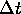,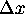,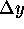,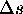),] [ANGLE=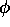,] [BETAGAMMA=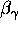,] [AXIS=(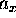,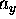,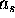),] [EV=(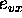,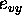,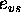),] [NOBEAM,] [RIGHT,] [LEFT,] [KIND=k(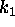,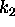),] [EXTERNALFIELD,] [LASER,] ;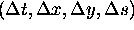Shift of origin. (m)Spacial rotation angle (radian). (rotation of the coordinate axis.)Lorentz boost parameter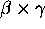. (Boost of the coordinate axis).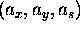Unit vector along the rotation axis. Need not be normalized exactly.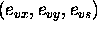Unit vector along the boost direction. Need not be normalized exactly.
NOBEAM
No transformation of particles. If specified, RIGHT, LEFT, KIND operands are ignored.
RIGHT,LEFT
Select right- or left-going particles only. If omitted, both are transformed.
k
Select only particles of kind k. If omitted, all species are transformed.
EXTERNALFIELD
Lorentz transformation of external field (transformation of the field strength and the boundary).
LASER
Lorentz transformation of lasers.

The three types of transformations are carried out in the order of the input keywords TXYS, ANGLE, BETAGAMMA. With one LORENTZ command, each transformation can be specified at most once.

Note that, for any type, the transformation is that of the coordinate axis rather than the particles themselves. Thus, for example, if you say TXYS=(0,0,0,1), then the s-coordinate of the particles by 1 meter.

Toshiaki Tauchi
Thu Dec 3 17:27:26 JST 1998